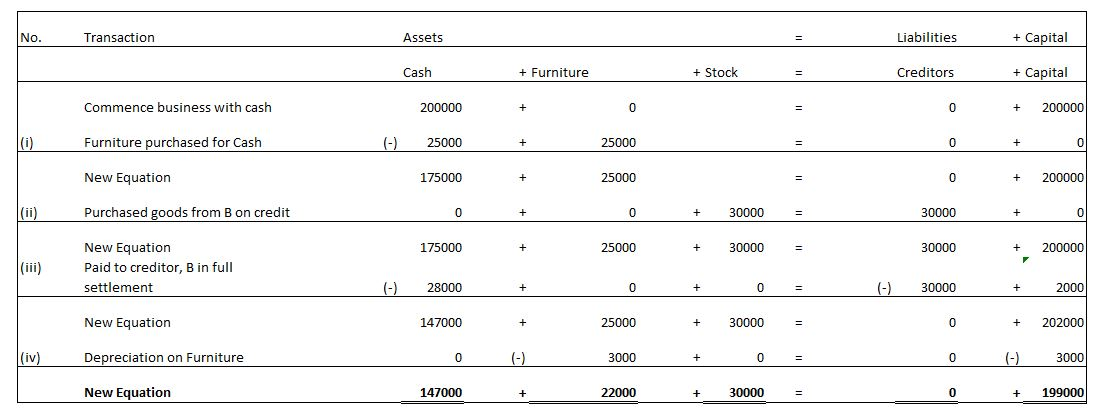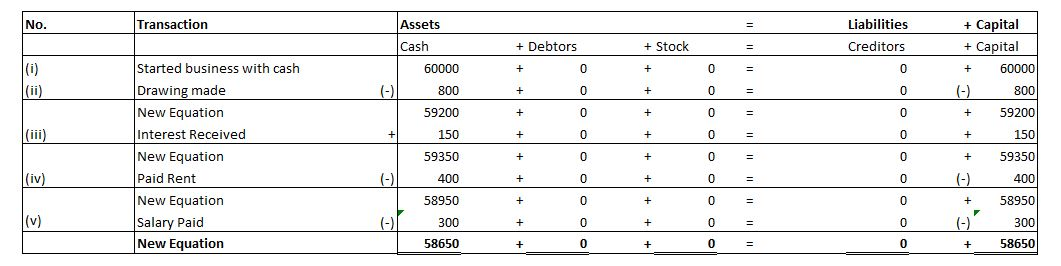# Accounting Equation

## What is Accounting Equation ?

Dual principle of accounting states that every transaction has two fold impact, i.e it impacts at least two accounts. Accounting equation for class 11, represents this dual principle of accounting, and it  is generally written as under : – Assets = Liabilities + Capital (Accounting equation Problems and Accounting Equation Question are dealt with in Class 11).

The accounting equation basically represents, that the Assets of the business, are always equal to the liabilities of the business to the outsiders (Liabilities), and the liabilities of the business to the Owners (“Capital”). Any transaction that a business may undertake, will always have an impact on the two sides in such a manner, that the equality of two sides is maintained at all times.

### Accounting Equation for Class 11 – Problems and Concept

The accounting equation concept has been explained in this video : –

### Impact on Purchase of Building in Cash

What would be the impact on Accounting Equation on Purchase of Building in Cash worth Rs. 150000 ?

Explanation:

Purchase of Building , results in an Increase in the value of the Building , which is an Asset. Hence it  results in an  Increase in Asset by Rs. 150, 000 .

Further Purchase of Building in Cash also results in Decrease in Cash ,which is an Asset. So it also results in Decrease in Asset by Rs. 150000

### Accounting Equation Problem  4 :

What would be the impact on Accounting Equation of Depreciation on Building amounting to Rs. 150000 at 20 % ?

Explanation:

Since, Depreciation on Building results in decrease in the value of Building (an Asset),  it results in decrease in value of Assets by Rs. 30000 .

Further,  Depreciation on Building is debited to the Profit and Loss Account , which reduces the profit. Since reduction in profit is a loss,  it is  reduced from the Capital A/c by Rs. 30000 .

Question 5 :

What would be the impact on Accounting Equation of Commission Received in Advance in Cash amounting to Rs. 10000 ?

Explanation:

Since, Commission Received in Advance in Cash results in Increase in Cash , and Cash  is an Asset, receipt of Commission  results in an Increase in Asset by Rs. 10000 .

Further, Commission Received in Advance in Cash, for work which is required to be done in future,  results in an Increase in Liability of the business by Rs. 10000 .

### Accounting Equation Problem Class 11 – Capital Introduced

Question 6:

What would be the impact on Accounting Equation of Introduction of Capital into business amounting to Rs. 110,000 ?

Explanation:

Introduction of Capital into business by way of cash would result in increase in cash , and cash  is an Asset. So this transaction would  increase an Assets by Rs. 110,000 .

Introduction of capital increases the capital base of the business, and hence it will result in increase in capital by Rs. 110000

Question 7:

What would be the  impact on AE of Commission Received in Advance in Cash amounting to Rs. 10,000 ?

Explanation:

Since, Commission Received in Advance in Cash results in Increase in Cash , and Cash  is an Asset, receipt of Commission  results in an Increase in Asset by Rs. 10000 .

Further, Commission Received in Advance in Cash, for work which is required to be done in future,  results in an Increase in Liability of the business by Rs. 10000 .

### Drawings

Question 8:

What would be the  impact on Accounting Equation of Rs. 12,000 Withdrawn for Personal Use ?

Explanation:

Rs. 12,000 withdrawn for Personal Use results in decrease in cash in business, and cash is an Asset. Hence it results in decrease in an Asset by Rs. 12,000 .

Further, when an owner withdraws  Rs. 12,000   for Personal Use , the amount owed by the business to owner  decrease by Rs. 12,000 and hence  Capital is reduced by Rs. 12,000 .

### Accounting Equation Class 11 – Interest Received

Question 9:

What would be the  impact on Accounting Equation of Interest Received  amounting to Rs. 1,500 ?

Explanation:

Interest of  Rs. 1,500 received by business  results in increase in cash , which is an Asset. Hence  it results in an increase in Asset by Rs. 1,500 .

Further, such interest increases the profit of the business and hence results in increase in capital , so the Capital is increased by Rs. 1,500

### Accounting Equation Question Class 11 – Rent Paid

Question 10:

What would be the  impact on AE of Maintenance Charges Paid in cash amounting to Rs. 3000 ?

Explanation:

Since, payment of maintenance Charges  amounting to Rs. 3000 , decrease in cash ,   it results in decrease in an Asset by Rs. 3000.

Further,  payment of Maintenance Charges  amounting  Rs. 3000,  results in a decrease in capital , and accordingly Capital is decreased by Rs. 3000.

### Accounting Equation Question Class 11 – Payment to Creditors

Question 11:

Mr. A started business as on April 1 , 2013 with a capital of Rs. 200000 . During the year, the following transactions took place:
(i) Furniture purchased for Cash Rs. 25000
(ii) Purchased goods from B on credit Rs. 30000 .
(iii) Paid to creditor, B ,Rs. 28000 in full settlement .
(iv) Depreciation on Furniture provided at 12 % .

Explanation:### Accounting Equation Class 11 – Drawings, Interest, Rent & Salary

Question 12:

Prepare Accounting Equation as per the details given below:
(i) A started business with cash Rs. 60000
(ii) Drawing made @ Rs. 800
(iii) Interest Received @ Rs. 150
(iv) Paid Rent @ Rs. 400
(v) Salary Paid @ Rs. 300

Explanation: